# Unconditional summability

(diff) ← Older revision | Latest revision (diff) | Newer revision → (diff)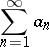(*)
is called unconditionally summable by some summation method(unconditionally-summable) if it is summable by this method to a sumwhatever the ordering of its terms, where the value ofmay depend on the particular rearrangement (cf. Summation methods). The study of unconditional summability originated with W. Orlicz ; he showed, in particular, that if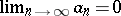, then absolute summability of the series by a linear regular method (cf. Regular summation methods) implies unconditional convergence. It was subsequently shown that this condition may be replaced by a weaker one: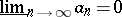. Unconditional summability by a matrix method does not imply unconditional convergence; in fact, take the series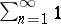. Ifis a regular matrix summation method and if the series (*) is unconditionally-summable, then all its terms have the form, whereis a constant and the series with terms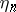is absolutely convergent: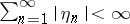; moreover,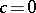if the methoddoes not sum the series.
In the case of series of functions one distinguishes between summability in measure, everywhere summability, almost-everywhere summability, etc. For unconditional summability of a series of functions, the following statement is valid almost-everywhere: If the series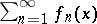of measurable functions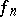on a setis unconditionally-summable almost-everywhere on, then the terms of this series have the form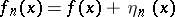, whereis a finite measurable function onand the series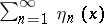is unconditionally almost-everywhere convergent on; also,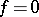ifdoes not sum.